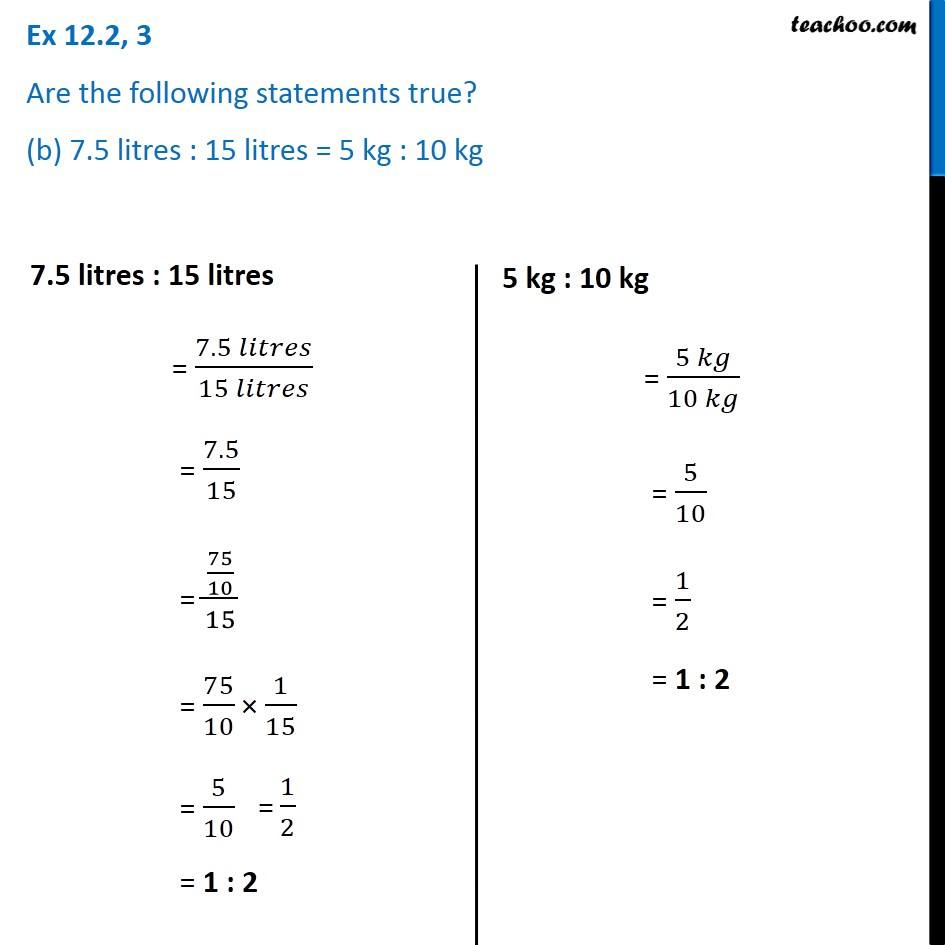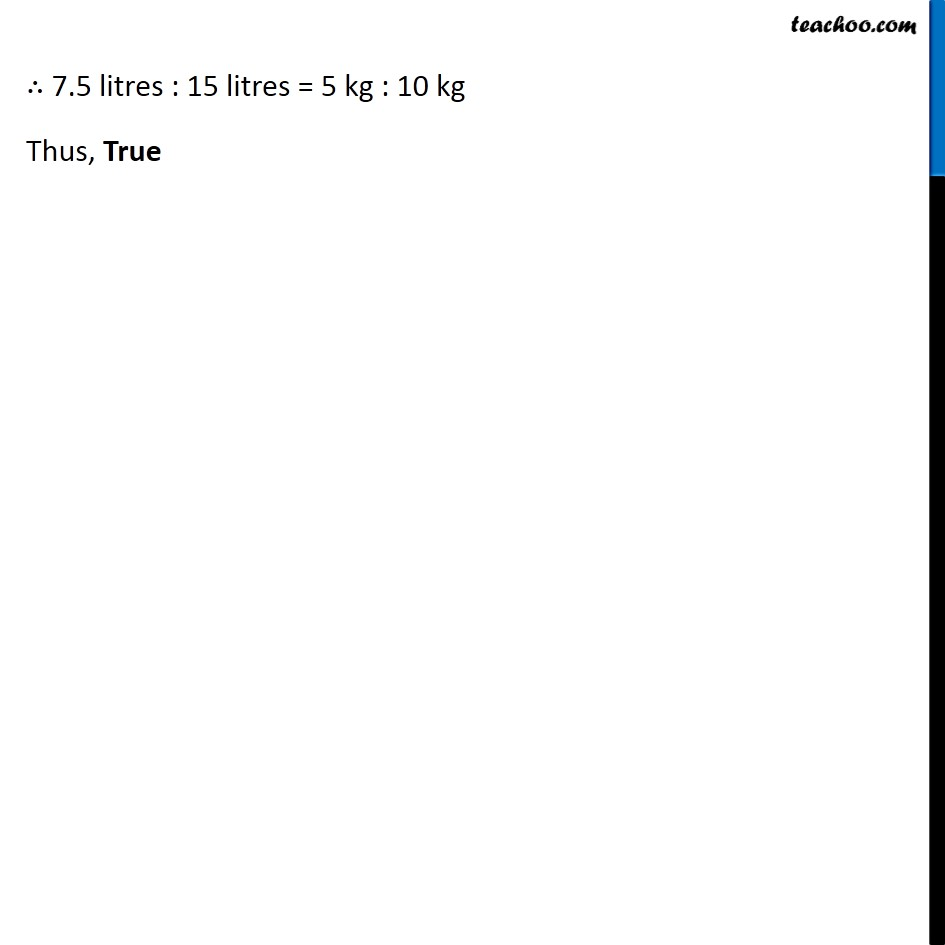Ex 12.2

Chapter 12 Class 6 Ratio and Proportion
Serial order wiseLearn in your speed, with individual attention - Teachoo Maths 1-on-1 Class

### Transcript

Ex 12.2, 3 Are the following statements true? (b) 7.5 litres : 15 litres = 5 kg : 10 kg7.5 litres : 15 litres = (7.5 𝑙𝑖𝑡𝑟𝑒𝑠)/(15 𝑙𝑖𝑡𝑟𝑒𝑠) = 7.5/15 = (75/10)/15 = 75/10 × 1/15 = 5/10 = 1 : 2 5 kg : 10 kg = (5 𝑘𝑔)/(10 𝑘𝑔) = 5/10 = 1/2 = 1 : 2 ∴ 7.5 litres : 15 litres = 5 kg : 10 kg Thus, True